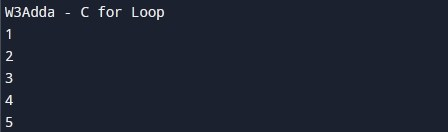# C for Loop

In this tutorial you will learn about the C for Loop and its application with practical example.

## C for Loop

The for loop executes a block of code repeatedly until a given condition evaluates to false. The C for loop is similar to the Java and C++ for loop. The for loop is used to repeat a block of code known number of times. When we want to execute a block of code for fixed number of times, it is recommended to use for loop. The for loop takes a variable as iterator and assign it with an initial value. It iterate through the loop body as long as the test condition is true. Once the loop statements are executed for current iteration, the iterator is updated with new value. If the test condition is still valid, we loop another time. When test condition return a false value, then the for loop is ended.

### For Loop flowchart

The flowchart image illustrates the working of for loop statement:### for Loop Syntax

The general for loop syntax in c programming is as follows:

Syntax :-

Above is the general syntax of a for loop. We just initialize iterator, check condition and then increment or decrement iterator value. The for loop has three components separated by semicolons.

initialization :- In this section is used to declare and initialize the loop iterator. This is the first statement to be executed and executed only once at the beginning.

condition :- Next, the test condition is evaluated before every iteration, and if it returns a true value then the loop body is executed for current iteration. If it returns a false value then loop is terminated. Then control jumps to the next statement just after the for loop.

incr/decr :- Once, the loop body is executed for current iteration then the incr/decr statement is executed to update the iterator value and if condition is evaluated again. If it returns a true value then the loop body is executed another time. If test condition returns a false value then loop is terminated and control jumps to the next statement just after the for loop.

### How for Loop Works In C

The for loop is an entry-controlled loop. In for loop, test condition is evaluated before processing the loop body. If a condition is true then and only then the body of a loop is executed. Once the loop body is executed then control goes back to the beginning, and the condition is checked again and if it is true, the same process is executed until the condition becomes false. Once the condition becomes false, the control goes out of the loop.

### Why We Need For Loop?

• Like any other loop “for loop” can also be used to execute the block of code over and over again.
• The advantage of a for loop is that we know exactly how many times the loop will be executed before the loop starts.

### For Loop Example In c

Below is the simple for loop example to demonstrate the use of for loop in c programming:

Example:-

Here, we have initialized ctr with initial value of 1, in the next we checks the condition ctr <= 5 for each of the iteration. If the test condition returns True, the statements inside loop body are executed and if test condition returns False then the for loop is terminated. In incr/decr section we have incremented the ctr value by one for each if the iteration. When we run the above C program, we see the following output –

Output:-## Various Forms of for loop In C

Let’s understand the various possible forms of for loop in c programming. Various forms of for loop are as follows:

### for loop without initialization

Initialization can be skipped from a for loop. Even though we can skip initialization part but semicolon (;) before test condition is must. If you missed semicolon (;), then you will get a compilation error.

Example:-

### for loop without increment or decrement

It is also possible to skip the increment or decrement part in for loop. In this case semicolon (;) is must after test condition. In this case the increment or decrement part is done inside the loop body.

Example:-

### for loop without initialization and without increment

In this for loop form, the loop variable can be initialized before the loop and can be increment or decremented inside the loop.

Example:-

### Multiple Initialization In for Loop

It is also possible to initialize multiple variables in for loop.

Example:-

Multiple test conditions can be joined with logical AND (&&) and/or OR (||) operators. It has two variables in increment part. Should be separated by comma.

In this tutorial we have learn about the C for Loop and its application with practical example. I hope you will like this tutorial.